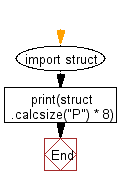﻿ Python: Determine if the python shell is executing in 32bit or 64bit mode on operating system - w3resource# Python: Determine if the python shell is executing in 32bit or 64bit mode on operating system

## Python Basic: Exercise-143 with Solution

Write a Python program to determine if the python shell is executing in 32bit or 64bit mode on operating system.

Sample Solution:-

Python Code:

``````import struct
print(struct.calcsize("P") * 8)
``````

Sample Output:

```64
```

Flowchart:Python Code Editor:

Have another way to solve this solution? Contribute your code (and comments) through Disqus.

What is the difficulty level of this exercise?

Test your Python skills with w3resource's quiz

﻿

## Python: Tips of the Day

List comprehension:

```>>> m = [x ** 2 for x in range(5)]
>>> m
[0, 1, 4, 9, 16]
```